7.2 Slider Crank Mechanisms

Another mechanism that has a very wide usage in machine design is the slider-crank mechanism. It is mainly used to convert rotary motion to a reciprocating motion or vice versa. Below a  she slider-crank mechanism is shown and the parameters that are used to define the angles and the link lengths are given.  As in the four-bar mechanism, the extended and folded dead centre positions are when the crank and the coupler are collinear (coupler link is commonly called  connecting rod  in slider-crank mechanisms). Full rotation of the crank is possible if the eccentricity, c, is less than the difference between the connecting rod and the crank lengths and the crank length is less than the connecting rod length (e.g. c<(a3-a2)  and a3>a2) .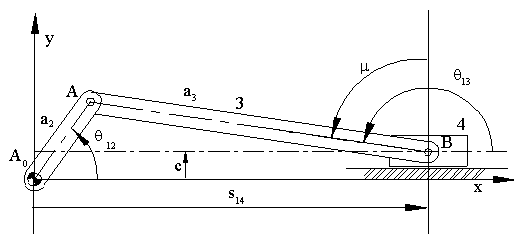Using the right angled triangles formed at the dead centre positions:Noting s =se-sf  =stroke = the distance slider travels between dead-centres.  If we let l= a2/a3 and e = c/a3 , the stroke will be given by: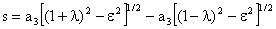If the eccentricity, c (or a1), is zero ( c = 0) the slider crank mechanism is called an in-line slider-crank  and the stroke is twice the crank length (s = 2a2). If the eccentricity is not zero ( c ¹0), it is usually called an offset slider-crank mechanism.

The transmission angle can be determined from the equation:

 a3cos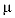=a2sin12-c (1)

Maximum deviation of the transmission angle occurs when the derivative of m with respect to q12 is zero.  Hence differentiating equation (1) with respect to q 12:(2)

Maximum or minimum deviation occurs when q 12  is 900 or 2700 (Fig. 7.19 ) and the value of the maximum or minimum transmission angle is given by:(3)

If c is positive as shown below, transmission angle is critical when q 12=2700. If c is negative, then the most critical transmission angle is at

q 12=900.If the eccentricity, c, is zero, maximum value of the transmission angle is:(4)

In reciprocating pumps, the crank-to-connecting rod ratio is kept less than 1/4, which corresponds to 14.480 maximum deviation of the transmission angle from 900. Since the crank length is fixed by the required stroke (a2 = s/2) one must increase the  connecting-rod length for better transmission angles. However, this will increase the size of the mechanism.

Similar to the transmission angle problem in the four-bar mechanisms, the transmission angle problem in slider-crank mechanisms can be stated as follows:

"Determine the slider-crank proportions with a given stroke, s, and corresponding crank rotation between dead-centers, f, such that the maximum deviation of the transmission angle from 900 is a minimum.”

The problem can again be considered in two parts. The first part is the determination of slider crank mechanisms with a given stroke and corresponding crank rotation. The second part is the determination of one particular slider-crank mechanism with optimum transmission angle variation.

For the first part of the problem note that the stroke s is a function of the link length ratios, i.e. if we double the length of the links, the stroke will be doubled. Therefore without loss of generality, let s=1 (the link lengths thus found will be multiplied by the stroke to give the actual values).

Referring to the figure where the slider crank mechanism is drawn at its dead centers, the vector loop equations at the dead centers are:(5)(6)

or in complex numbers: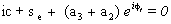(7)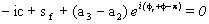(8)

Subtracting eq.(8)  from  eq. (7)  and noting  se-sf = s = 1 :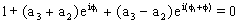(9)

If  we let Z =and  l = a2/a3  equation (8) can be rewritten in the form: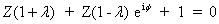(10)

For a full rotation of the crank a necessary (but not sufficient)  condition  is l<1. Equation 10 can be solved for Z to yield:(11)

If l is taken as a free parameter, as it varies, the tip of Z given by (7), will generate a circle which is the locus of all possible moving pivots for the crank when the crank and the coupler are at extended position (ka circle). The locus of all possible fixed pivots is another circle  (k0 circle) which is given by Z(1+l) (the origin for both vectors is Be with the real axis parallel to the slider axis).. Any line drawn from Be intersects these circles at Ae and A0 respectively, yielding the slider-crank mechanism in extended dead centre position. Below these circles are shown for f=1600.The eccentricity, c can be obtained as the imaginary component of the vector BeA0=BeAe+AeA0 which can be written as: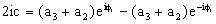(12)

or using Z and  l: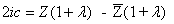(13)

and substituting the value of Z:(14)

The link lengths can now be expressed as: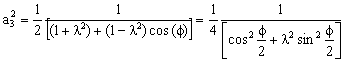(15)(16)

Equations (14-16) yield a singly infinite set of solutions for the slider-crank mechanisms satisfying a given crank rotation (stroke = 1 unit). One can also use the eccentricity, crank or coupler link length as the free parameter to determine the other link lengths.

For the Geometric Solution:

Example 4.6:

Determine the link lengths of the slider crank mechanism with a stoke s=120 mm, corresponding crank rotation f=1600 and the crank to coupler link ratio l=0.5.

Using unit stroke, from equations (14), (15) and (16) the link lengths are:

a2 = 0.47881,     a3= 0.95762   and   c = 0.23523.  For   s = 120:

a2= 114.91mm,      a3 = 57.46mm  and c = 28.23mm.

The minimum transmission angle for this mechanism is mmin = 41.790.

Example 4.7:

Determine the link lengths of the slider-crank mechanism having the same stoke and corresponding crank rotation as in example 1 but instead of crank to coupler link ratio specified, the eccentricity is specified as c = 20 mm.

For unit stroke c = 20/120  =  0.16667. Solving equation (10) for l yields:(17)

For c = 0.16667, l2 = 0.325635. Substituting into equations (15) and (16),  a2 = 0.48508 and a3 = 0.85006. For s= 120 mm,  c = 20mm ,   a2 = 58.21 mm and  a3 = 102.01mm. The minimum transmission angle for this mechanism is mmin= 39.940. Note that a similar procedure can be carried out if crank or coupler link length is specified.

The minimum transmission angle is when q = p/2:(18)

For the full rotatability of the crank, c+ a2 < a3  or  c < a3-a2. At the limit position (c = a3- a2),  mmin = 0.  Using equation (14), (15) and (16) this condition yields the limits of f for crank rotatability as:and                                  (19)Expressing mmin in terms of l and f (substitute eqs 14,15 and 16 into eq.18 and simplify)(20)

since l is a free design parameter, the necessary condition for the minimum transmission angle to be a maximum is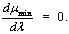If the value of l which makes the derivative equal to zero is  l = lopt, differentiating equation (20) and settingyields.(21)

Where Q = l2optt2  and  t = tan(f/2).  The roots of equation (21) are: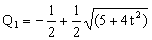(22)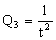Since Q must be positive, Q>2 is not a solution. Corresponding to Q3, l=1/t2, the deviation of the minimum transmission angle 900 is maximized ( cosmmin=1). The root Q1 yields the value of lopt  within the range (1/t2, l), and this value satisfies the necessary and sufficient condition for slider-crank mechanism with optimum transmission angle characteristics. Therefore:(23)
is the unique optimum solution.

Example 4.8:
For slider stroke s=120 mm and corresponding crank rotation f=1600, determine the slider crank mechanism with optimum force transmission characteristics.

From equation (20),. Utilising equations (14), (15) and (16) for unit stroke the link lengths are a2= 0.465542  ;  a3= 1.14896 ; c= 0.377378 and for 120 mm stroke:

a2= 55.87 mm; a3= 137.88 mm ; c= 42.81 mm

The minimum transmission angle for the mechanism is  mmin= 42.810.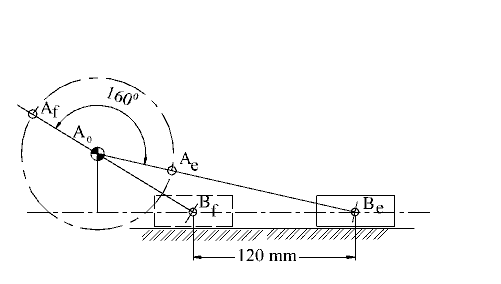The results are given in Chart 2. The slider-crank link lengths (a2, a3, c) and optimum values and the minimum transmission angle mmin as function of crank rotation between dead centres is given. In Chart 3, all the possible solutions and their minimum transmission angle values are given (note that the horizontal axis is not to a linear scale).